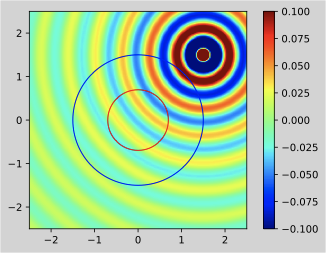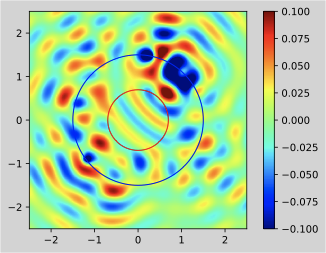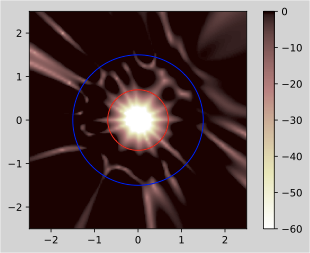# Mode matching method

## What is the mode matching method?

The mode matching method is a method that aims to match the mode of the desired sound field synthesized at a specific control point. Since it is a numerical solution, there are no restrictions on the placement of speakers, but it can be numerically unstable. In this article, I would like to write a simulation when the speaker arrangement is made spherical by the mode matching method. Higher-order Ambisonics simulation The sound field is reproduced by minimizing the squared error of the expansion coefficient described in the article.

The functions used in the mode matching method are as follows. In addition, the function of green_function is described in the above article of Higher Ambisonics.

#### function.py


import scipy as sp
from scipy.special import sph_harm
import numpy as np
from scipy.special import spherical_jn
from scipy.special import spherical_yn
from numpy import arctan2, sqrt
import numexpr as ne

def cart2sph(x,y,z):
""" x, y, z :  ndarray coordinates
ceval: backend to use:
- eval :  pure Numpy
- numexpr.evaluate:  Numexpr """

azimuth = ne.evaluate('arctan2(y,x)')
xy2 = ne.evaluate('x**2 + y**2')
elevation = ne.evaluate('arctan2(z, sqrt(xy2))')
r = eval('sqrt(xy2 + z**2)')
return azimuth, elevation, r

def EquiDistSphere(n):
golden_angle = np.pi * (3 - np.sqrt(5))
theta = golden_angle * (np.arange(n)+1)
z = np.linspace(1 - 1.0 / n, 1.0 / n - 1, n)
radius = np.sqrt(1 - z * z)

points = np.zeros((n, 3))
points[:,0] = radius * np.cos(theta)
points[:,1] = radius * np.sin(theta)
points[:,2] = z
return points

def BnMat2(N,k,r):
B = np.zeros((N+1)**2, dtype = np.complex).reshape((N+1)**2,)
dx = 0
for i in range(N+1):
A = Bn2(i,k,r)
for j in np.arange(-i,i+1,1):
B[dx] = A
dx +=1
return B

def Bn2(n,k,r):
kr = k*r
y = shankel(n,1,kr)
return y

def shankel(n,k,z):
if k == 1:
sha = spherical_jn(n,z) + 1j*spherical_yn(n,z)
else:
sha = spherical_jn(n,z) - 1j*spherical_yn(n,z)
return sha

def calcSH(N,azi,ele):
y_n = np.array([],dtype = np.complex)
for n in range(N+1):
for m in np.arange(-n,n+1,1):
y_n = np.append(y_n,sph_harm(m,n,azi,ele))
return y_n

def calcCmn(N,k,r,pos):
C_N = np.zeros(((N+1)**2,int(len(pos))),dtype = np.complex)
if len(pos) == 1:
hn = BnMat2(N,k,r)
C_N = hn * np.conj(calcSH(N,pos[0,0],pos[0,1])).T
else:
hn = BnMat2(N,k,r)
for i in range(int(len(pos[:,0]))):
C_N[:,i] = hn * np.conj(calcSH(N,pos[i,0],pos[i,1])).T

return C_N


## simulation

In this simulation as well, we will consider using spherical waves.

\frac{e^{ik|r-r_s|}}{4\pi|r-r_s|} = \sum_{n=0}^\infty\sum_{m=-n}^n ik j_n(kr) h_n(kr_s) Y_n^m(\theta,\varphi) Y_n^m(\theta_s,\varphi_s)^*

The sound pressure on the sphere can be expressed as follows. $p\bigl(\theta,\varphi,r,\omega\bigr) = \sum_{n=0}^\infty\sum_{m=-n}^n A_{nm} 4\pi i^n j_n(kr) Y_n^m(\theta,\varphi)$

From these two formulas $A_{nm} = \frac{k h_n(kr_s) Y_n^m(\theta_s,\varphi_s)^* }{4\pi i^{n-1}}$

The expansion coefficient can be obtained. The expansion coefficient of the desired sound field and the expansion coefficient of the reproduced sound field can be written respectively.

A_{nm}^{des} = \frac{k h_n(kr_{s1}) Y_n^m(\theta_{s1},\varphi_{s1})^*}{4\pi i^{n-1}}
A_{nm}^{rep} = \frac{k h_n(kr_{s2}) Y_n^m(\theta_{s2},\varphi_{s2})^*d}{4\pi i^{n-1}}

$s1$ and $s2$ represent the primary and secondary sound sources. The problem is to find a drive signal that minimizes this squared error.

min {|A_{nm}^{rep} - A_{nm}^{des}|}^2 + \lambda d^H d

If you expand this formula $C = h_n(kr_{s2}) Y_n^m(\theta_{s2},\varphi_{s2})^*$ $b = h_n(kr_{s1}) Y_n^m(\theta_{s1},\varphi_{s1})^*$ $d = \Bigl(C^H C + \lambda I\Bigr)^{-1} + C^H b$

More drive signals can be obtained.

#### mode_matching_method.py


import green_function as gr
import numpy as np
import scipy as sp
import function as fu
import matplotlib.pyplot as plt
import matplotlib.patches as patches
import matplotlib.cm as cm

pos = np.array([0,0,0])

#Initial conditions
freq = 550
cv = 344
num_sp = 64
lambda_ = 10**-8
r_sp = 1.5

#Graph conditions
Plot_range = 2.5
space = 0.05

#Calculation
omega = 2 * np.pi * freq
k = omega/cv
pos_azi, pos_ele, pos_r = fu.cart2sph(pos,pos,pos)
vec_R = np.array([pos_azi, pos_ele, pos_r])

#Speaker position
sp_xyz = fu.EquiDistSphere(num_sp)
sp_xyz = sp_xyz * r_sp
sp_azi,sp_ele, sp_r = fu.cart2sph(sp_xyz[:,0],sp_xyz[:,1],sp_xyz[:,2])
sp_ele1 = np.pi/2 - sp_ele
sp_sph = np.vstack([np.vstack([sp_azi,sp_ele1]),sp_r]).T

#Optimal order determination
N_q = int(np.floor(np.sqrt(num_sp))-1)

#Primary sound source
ps_xyz = np.array([1.5,1.5,0]) #Place the radius outside the speaker placement
ps_azi,ps_ele, ps_r = fu.cart2sph(ps_xyz,ps_xyz,ps_xyz)
ps_ele1 = np.pi/2 - ps_ele
ps_sph = np.vstack([np.vstack([ps_azi,ps_ele1]),ps_r]).T

#Calculation of mode matching method
C = fu.calcCmn(N_q,k,r_sp,sp_sph);
b = fu.calcCmn(N_q,k,ps_sph[0,2],ps_sph);

I = np.identity(num_sp)
CC = np.conj(C.T) @ C
Cb = np.conj(C.T) @ b

d_MM = 	np.linalg.solve(CC + lambda_ * I,Cb)

#Desired sound field(Primary sound source)And seek the reproduction sound field
X = np.arange(-Plot_range,Plot_range+space,space)
Y = np.arange(-Plot_range,Plot_range+space,space)
P_org = np.zeros((X.size,Y.size), dtype = np.complex)
MM = np.zeros((X.size,Y.size), dtype = np.complex)
for j in range(X.size):
for i in range(Y.size):
pos_r = np.array([X[j],Y[i],0])
P_org[i,j] = gr.green2(1,freq,cv,ps_xyz,pos_r)
for l in range(num_sp):
G_transfer = gr.green2(1,freq,cv,sp_xyz[l,:],pos_r)
MM[i,j] += G_transfer *  d_MM[l]

NE = np.zeros((X.size,Y.size), dtype = np.complex)
for i in range(X.size):
for j in range(Y.size):
NE[i,j] = 10*np.log10(((np.abs(MM[i,j]-P_org[i,j]))**2)/((np.abs(P_org[i,j]))**2))

#Sweet spot

#Graph
#Desired sound field
r_sp_ = patches.Circle(xy=(0, 0), radius=r_sp, ec='b',fill=False)
plt.figure()
ax = plt.axes()
im = ax.imshow(np.real(P_org), interpolation='gaussian',cmap=cm.jet,origin='lower',
extent=[-Plot_range, Plot_range, -Plot_range, Plot_range],
vmax=0.1, vmin=-0.1)
ax.grid(False)
plt.axis('scaled')
ax.set_aspect('equal')
plt.colorbar(im)
plt.savefig('original_sound.pdf',bbox_inches="tight",dpi = 64,
facecolor = "lightgray", tight_layout = True)
plt.show()

#Reproduced sound field
r_sp_ = patches.Circle(xy=(0, 0), radius=r_sp, ec='b',fill=False)
plt.figure()
ax2 = plt.axes()
im2 = ax2.imshow(np.real(MM), interpolation='gaussian',cmap=cm.jet,origin='lower',
extent=[-Plot_range, Plot_range, -Plot_range, Plot_range],
vmax=0.1, vmin=-0.1)
ax2.grid(False)
plt.axis('scaled')
ax2.set_aspect('equal')
plt.colorbar(im2)
plt.savefig('reproduced_sound.pdf',bbox_inches="tight",dpi = 64,
facecolor = "lightgray", tight_layout = True)
plt.show()

#Normalization error
r_sp_ = patches.Circle(xy=(0, 0), radius=r_sp, ec='b',fill=False)
plt.figure()
ax3 = plt.axes()
im3 = ax3.imshow(np.real(NE), interpolation='gaussian',cmap=cm.pink_r,origin='lower',
extent=[-Plot_range, Plot_range, -Plot_range, Plot_range],
vmax=0, vmin=-60)
ax3.grid(False)
plt.axis('scaled')
ax3.set_aspect('equal')
plt.colorbar(im3)
plt.savefig('normalization_error.pdf',bbox_inches="tight",dpi = 64,
facecolor = "lightgray", tight_layout = True)
plt.show()



## result

Desired sound fieldReproduced sound fieldNormalization error## Summary

It was a spherical arrangement ver of the mode matching method. I have the code on Github. https://github.com/koseki2580/mode_matching_method